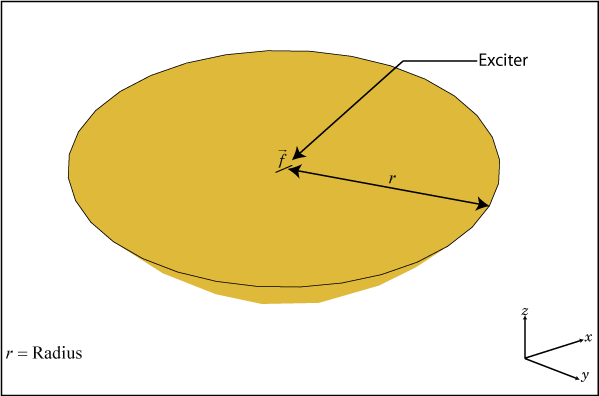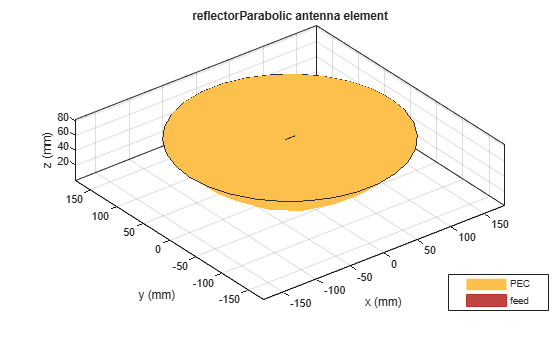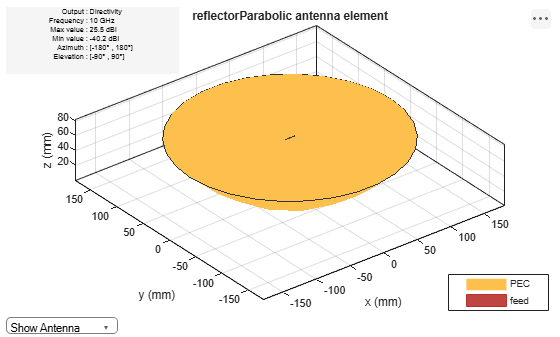# reflectorParabolic

Create parabolic reflector antenna

## Description

The `reflectorParabolic` object creates a parabolic reflector antenna. Parabolic reflector antennas are electrically large structures and are at least 10 wavelengths in diameter. These reflectors are used in TV antennas and satellite communications, for example.## Creation

### Syntax

``ant = reflectorParabolic``
``ant = reflectorParabolic(Name=Value)``

### Description

example

````ant = reflectorParabolic` creates a dipole-fed parabolic reflector antenna. The default antenna exciter operates at 10 GHz. The reflector is 10λ in diameter, where λ corresponds to the value of wavelength.```
````ant = reflectorParabolic(Name=Value)` creates a parabolic reflector antenna, with additional Properties specified by one or more name–value arguments. `Name` is the property name and `Value` is the corresponding value. You can specify several name-value arguments in any order as `Name1`= `Value1`, `...`, `NameN`=`ValueN`. Properties not specified retain their default values.For example, `ant = reflectorParabolic(FocalLength=0.5)` creates a parabolic reflector antenna with 0.5 meters focal length.```

## Properties

expand all

Exciter antenna or array type, specified as a single-element antenna object, an array object, measured pattern data of an antenna, or an empty array. Except for reflector and cavity antenna elements, you can use any Antenna Toolbox™ antenna or array element as an exciter. To create the cavity backing structure without the exciter, specify this property as an empty array.

Example: `horn`

Example: `linearArray(Element=patchMicrostrip)`

Example: `measuredAntenna`

Example: `[]`

Radius of the parabolic reflector, specified as a positive scalar in meters.

Example: `0.22`

Data Types: `double`

Focal length of the parabolic reflector, specified as a positive scalar in meters.

Example: `0.0850`

Data Types: `double`

Signed distance from the focus of the parabolic reflector, specified as a three-element vector in meters. By default, the antenna exciter is at the focus of the parabola. Using the `FeedOffset` property, you can place the exciter anywhere on the parabola.

Example: `[0.0850 0 0]`

Data Types: `double`

Type of the metal used as a conductor, specified as a metal material object. You can choose any metal from the `MetalCatalog` or specify a metal of your choice. For more information, see `metal`. For more information on metal conductor meshing, see Meshing.

Example: `metal("Copper")`

Lumped elements added to the antenna feed, specified as a lumped element object. For more information, see `lumpedElement`.

Example: `Load=lumpedelement`. `lumpedelement` is the object for the load created using `lumpedElement`.

Example: `lumpedElement(Impedance=75)`

Tilt angle of the antenna in degrees, specified as a scalar or vector. For more information, see Rotate Antennas and Arrays.

Example: `90`

Example: `Tilt=[90 90]`,`TiltAxis=[0 1 0;0 1 1]` tilts the antenna at 90 degrees about the two axes defined by the vectors.

Data Types: `double`

Tilt axis of the antenna, specified as one of these values:

• Three-element vector of Cartesian coordinates in meters. In this case, each coordinate in the vector starts at the origin and lies along the specified points on the x-, y-, and z-axes.

• Two points in space, specified as a 2-by-3 matrix corresponding to two three-element vectors of Cartesian coordinates. In this case, the antenna rotates around the line joining the two points.

• `"x"`, `"y"`, or `"z"` to describe a rotation about the x-, y-, or z-axis, respectively.

Example: `[0 1 0]`

Example: `[0 0 0;0 1 0]`

Example: `"Z"`

Data Types: `double` | `string`

Solver for antenna analysis, specified as a string. Default solver is `"MoM-PO"`(Method of Moments-Physical Optics hybrid). Other supported solvers are: `"MoM"` (Method of Moments), `"PO"` (Physical optics) or `"FMM"` (Fast Multipole Method).

Example: `SolverType`=`"MoM"`

Data Types: `string`

## Object Functions

 `show` Display antenna, array structures, shapes, or platform `solver` Access FMM solver for electromagnetic analysis `axialRatio` Axial ratio of antenna `beamwidth` Beamwidth of antenna `charge` Charge distribution on antenna or array surface `current` Current distribution on antenna or array surface `design` Design prototype antenna or arrays for resonance around specified frequency or create AI-based antenna from antenna catalog objects `efficiency` Radiation efficiency of antenna `EHfields` Electric and magnetic fields of antennas or embedded electric and magnetic fields of antenna element in arrays `impedance` Input impedance of antenna or scan impedance of array `mesh` Mesh properties of metal, dielectric antenna, or array structure `meshconfig` Change meshing mode of antenna, array, custom antenna, custom array, or custom geometry `optimize` Optimize antenna or array using SADEA optimizer `pattern` Plot radiation pattern and phase of antenna or array or embedded pattern of antenna element in array `patternAzimuth` Azimuth plane radiation pattern of antenna or array `patternElevation` Elevation plane radiation pattern of antenna or array `rcs` Calculate and plot radar cross section (RCS) of platform, antenna, or array `returnLoss` Return loss of antenna or scan return loss of array `sparameters` Calculate S-parameters for antennas and antenna arrays `vswr` Voltage standing wave ratio (VSWR) of antenna or array element

## Examples

collapse all

Create and view a default parabolic reflector antenna.

`ant = reflectorParabolic`
```ant = reflectorParabolic with properties: Exciter: [1x1 dipole] Radius: 0.1500 FocalLength: 0.0750 FeedOffset: [0 0 0] Tilt: 0 TiltAxis: [1 0 0] Load: [1x1 lumpedElement] SolverType: 'MoM-PO' ```
`show(ant)`Plot the radiation pattern of the parabolic reflector at 10 GHz.

`pattern(ant,10e9)`Create a circular array of equiangular spiral antennas.

`circA = circularArray(Element=spiralEquiangular,Radius=0.1);`

Create a parabolic reflector-backed antenna.

`ant = reflectorParabolic(Exciter=circA)`
```ant = reflectorParabolic with properties: Exciter: [1x1 circularArray] Radius: 0.1500 FocalLength: 0.0750 FeedOffset: [0 0 0] Tilt: 0 TiltAxis: [1 0 0] Load: [1x1 lumpedElement] SolverType: 'MoM-PO' ```
`show(ant)`## Version History

Introduced in R2018b